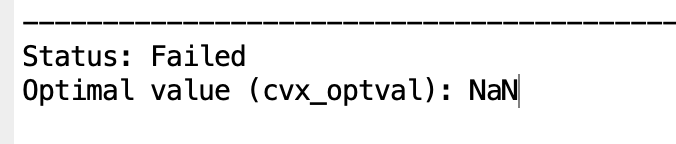# Status failed but with results

I am running below codes by cvx toolbox:

cvx_begin
variable w(M*J,1) complex
minimize max(abs(C_clo’*w))
subject to
for i = 1:subband
abs(Ck(:,i)’*w) <= 0.01;
end
real(C0’*w) == f0;
cvx_end

However, the status shown failed. I verified both of the constraints and found that the second one (real(C0’*w) == f0) seems not satisfied by logical operation, while the difference could be very slight as shown in the graph. May I know in this situation can the results be used?

I will be appreciated if anyone can help with this!

We don’t know what you mean by `Status failed ` because you haven’t shown the CVX and solver output.

Even if the solver and CVX claim to have succeeded in finding an optimum, (equality) constrains only need be satisfied to within a feasibility tolerance. Therefore, you can’t expect equality constraints be satisfied as logical equalities The results you displayed showed those elements of the equality constraint are satisfied to at least 4 decimal places.

See http://cvxr.com/cvx/doc/solver.html#controlling-precision . I recommend you keep tolerances at default values.

Hi Mark,

Very thanks for your reply. My result is shown as below. That might be the issue of precision. I will set the precision as “low” and check again. Thanks for your suggestion!You still haven’;t shown the solver output.

And I did not recommend changing the precision from the default. I recommended keeping it at the default.

Hi Mark,
Below is my output. Hope you could give me some advice. Many thanks!

## Calling SDPT3 4.0: 5369 variables, 1723 equality constraints For improved efficiency, SDPT3 is solving the dual problem.

num. of constraints = 1723
dim. of socp var = 3966, num. of socp blk = 1322
dim. of linear var = 1323
dim. of free var = 80 *** convert ublk to lblk

SDPT3: Infeasible path-following algorithms

## number of iterations = 28 primal objective value = -9.93035860e-01 dual objective value = -1.00247094e+00 gap := trace(XZ) = 9.60e-03 relative gap = 3.21e-03 actual relative gap = 3.15e-03 rel. primal infeas (scaled problem) = 1.94e-05 rel. dual " " " = 4.05e-05 rel. primal infeas (unscaled problem) = 0.00e+00 rel. dual " " " = 0.00e+00 norm(X), norm(y), norm(Z) = 5.3e+01, 1.2e+03, 3.1e+01 norm(A), norm(b), norm© = 7.4e+02, 2.0e+00, 7.3e+00 Total CPU time (secs) = 19.63 CPU time per iteration = 0.70 termination code = -5 DIMACS: 1.9e-05 0.0e+00 1.5e-04 0.0e+00 3.1e-03 3.2e-03

Status: Failed
Optimal value (cvx_optval): NaN

Maybe your problem is near infeasible and therefore giving SDPT3 a hard time.

I would try Sedumi and/or Mosek to see what happens since they are better at dealing with infeasible problems.

I am surprised if the CVX variable `w` had any value other than `NaN` after the solver failed. In any event, the values of the CVX variables should be considered to be meaningless after the solver fails, and should not be used for anything.